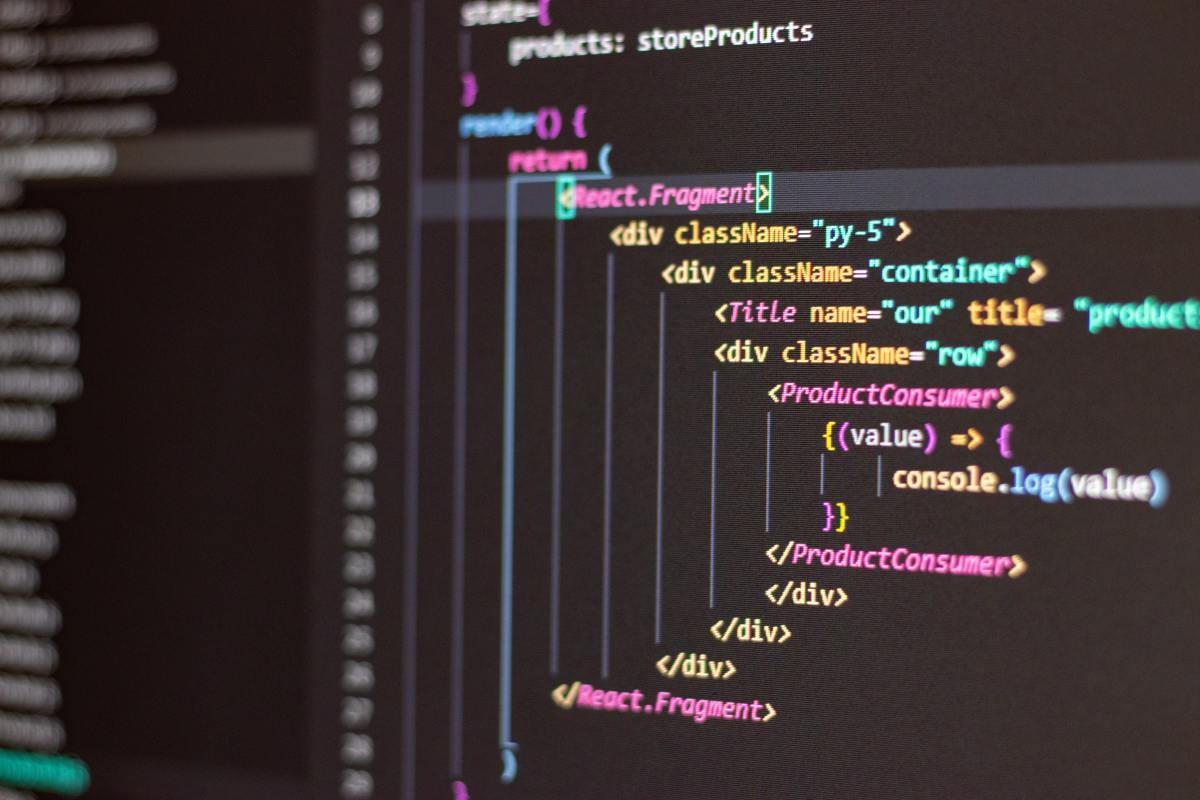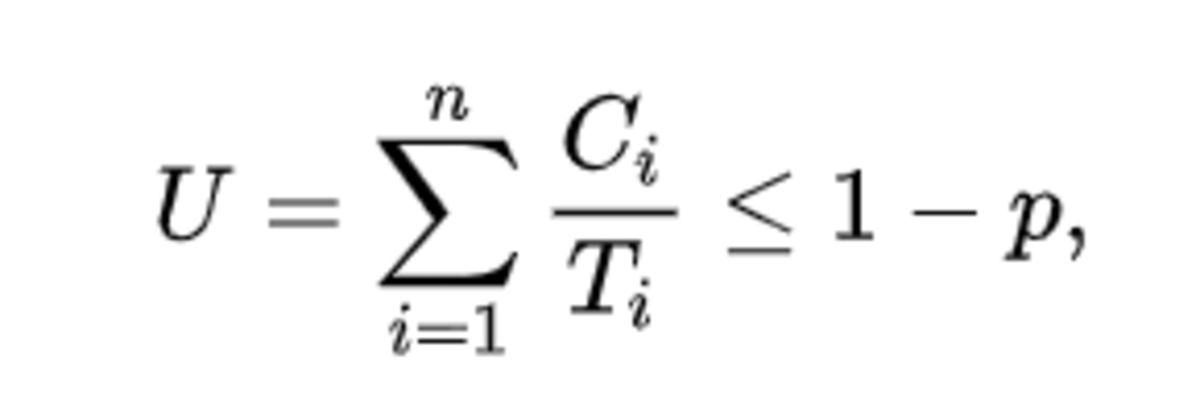# Scheduling Algorithms in Operating Systems Using Earliest Deadline First (EDF)

Arwa writes about programming and coding issues, including how to schedule algorithms in real-time operating systems.Scheduling algorithms are methods used in operating systems to distribute resources among processes in a scheduled manner.Photo by Antonio Batinić on Pexels

Scheduling algorithms are methods used in operating systems to distribute resources among processes in a scheduled manner. The purpose of these algorithms is to minimize resource starvation by ensuring that each process gets its required CPU time.

This article will explain one example of a scheduling algorithm used in real-time operating systems and earliest deadline first (EDF) scheduling.

## What Is EDF Scheduling?

Earliest deadline first (EDF) is an optimal dynamic priority scheduling algorithm mainly used in real-time operating systems. It can be described through the following points:

1. Priority driven: Each process is assigned a priority and the scheduler selects the process to run according to it. Hence, the process with the highest priority is carried out first. In the case of EDF, the priority is set according to the absolute deadline of each process.
2. Dynamic: Priorities are calculated and can change while the processes are running; unlike static scheduling, in which the priorities are already assigned before the processes are being carried out.
3. Executes in preemptive mode: This means that time slots of the CPU can be divided for a process. In other words, it is not required that the same process holds the resource given to it throughout its life cycle. Instead, it can be interrupted by another process if that other process has a higher priority. EDF also runs on non-preemptive uniprocessors, but it does not allow inserted idle time.

## How EDF Works

Each process has an absolute deadline assigned to it and the scheduler runs the processes based on those deadlines. The process with the closest deadline is assigned the highest priority. The priorities are assigned and changed dynamically.

To ensure that a set of processes is schedulable using EDF, the following formula is used:where U is the CPU Utilization, Ci is the execution time, and Ti is the period for each process.

EDF can guarantee that all deadlines are met given that their utilization is less than or equal to 100%.

EDF has some advantages compared to fixed-priority scheduling algorithms. For instance, it has less context switches, less idle times (CPU can be fully utilized), and it can schedule all sets of processes that fixed-priority algorithms can, while EDF schedulable process are not all schedulable with fixed-priority algorithms.

However, there are a few limitations. For example, it is less predictable because of the variable priorities of the tasks, which might affect the system when it is overloaded. It is also less controllable in terms of priorities and execution. In addition, EDF has high overhead and is difficult to implement in hardware.

Scroll to Continue

## Example 1

Consider the three following processes:

3

2

2

7

4

8

20

5

10

### Step 1

First, we will check whether the processes are schedulable by calculating the utilization:
U = C1/P1 + C2/P2 + C3/P3 = (3/20) + (2/5) + (2/10) = 0.75 = 75%
U = 75 < 100%, which indicates that the processes are schedulable.

### Step 2

We take the least common multiple of the periods of the processes to know how many units we need to execute the three processes:
Lcm(20, 5, 10) = 20
We need 20 units at most to execute the processes.

### Step 3

Because the period of P1 = 10, we give it 20/10 = 2 time slices, each is 10 units long. Similarly, with P2 we get 20/5 = 4 time slices, each with a length of 5 units, and P3 will be given 20/20 = 1 time slice with a length of 20 units. The dividers are marked in green and shown in the figure below:

### Step 4

The process with the nearest deadline is given the priority to run. When its execution time is completed for that time slice, the next process with the nearest deadline will run. In this case, the deadlines are equal to the periods. this is illustrated below:

## Example 2

In this example, each of the processes from the previous example is given a deadline that is not equal to its period. We split the time given to each process as done in the previous example. However, in this case, we mark the deadline as follows (the deadlines are marked in black):